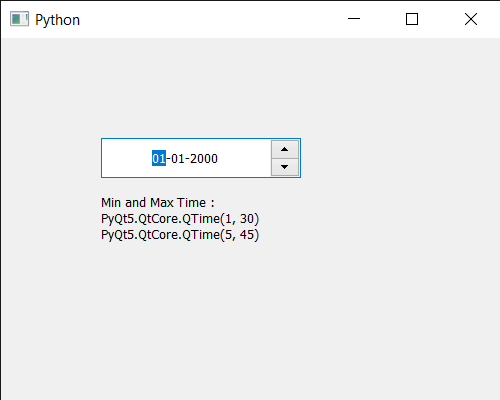# PyQt5 QDateEdit – Setting Time range which can be set

In this article we will see how we can set time range to the QDateEdit. Sometimes there is a need to set time range so that user can’t enter/store time which are beyond the time range. We can set time to date edit with the help of `setTime` method. Minimum and maximum time can be set to the date edit with the help of `setMinimumTime` and `setMaximumTime` method.

In order to do this we use `setTimeRange` method with the QDateEdit object

Syntax : date.setTimeRange(t1, t2)

Argument : It takes two QTime object as argument

Return : It returns None

Below is the implementation

 `# importing libraries ` `from` `PyQt5.QtWidgets ``import` `*`  `from` `PyQt5 ``import` `QtCore, QtGui ` `from` `PyQt5.QtGui ``import` `*`  `from` `PyQt5.QtCore ``import` `*`  `import` `sys ` ` `  ` `  `class` `Window(QMainWindow): ` ` `  `    ``def` `__init__(``self``): ` `        ``super``().__init__() ` ` `  `        ``# setting title ` `        ``self``.setWindowTitle(``"Python "``) ` ` `  `        ``# setting geometry ` `        ``self``.setGeometry(``100``, ``100``, ``500``, ``400``) ` ` `  `        ``# calling method ` `        ``self``.UiComponents() ` ` `  `        ``# showing all the widgets ` `        ``self``.show() ` ` `  `    ``# method for components ` `    ``def` `UiComponents(``self``): ` ` `  `        ``# creating a QDateEdit widget ` `        ``date ``=` `QDateEdit(``self``) ` ` `  `        ``# setting geometry of the date edit ` `        ``date.setGeometry(``100``, ``100``, ``200``, ``40``) ` ` `  `        ``# alignment ` `        ``a_flag ``=` `Qt.AlignCenter ` ` `  `        ``# setting alignment of date ` `        ``date.setAlignment(a_flag) ` ` `  `        ``# QTime object ` `        ``t1 ``=` `QTime(``1``, ``30``) ` `        ``t2 ``=` `QTime(``5``, ``45``) ` ` `  `        ``# setting minimum time ` `        ``date.setTimeRange(t1, t2) ` ` `  `        ``# creating a label ` `        ``label ``=` `QLabel(``"GeeksforGeeks"``, ``self``) ` ` `  `        ``# setting geometry ` `        ``label.setGeometry(``100``, ``150``, ``250``, ``60``) ` ` `  `        ``# making label multiline ` `        ``label.setWordWrap(``True``) ` ` `  `        ``# getting time ` `        ``value1 ``=` `date.minimumTime() ` `        ``value2 ``=` `date.maximumTime() ` ` `  ` `  `        ``# setting text to the label ` `        ``label.setText(``"Min and Max Time : "` `+` `str``(value1) ``+` `" "` `+` `str``(value2)) ` ` `  ` `  `# create pyqt5 app ` `App ``=` `QApplication(sys.argv) ` ` `  `# create the instance of our Window ` `window ``=` `Window() ` ` `  `# start the app ` `sys.exit(App.``exec``()) `

Output :Whether you're preparing for your first job interview or aiming to upskill in this ever-evolving tech landscape, GeeksforGeeks Courses are your key to success. We provide top-quality content at affordable prices, all geared towards accelerating your growth in a time-bound manner. Join the millions we've already empowered, and we're here to do the same for you. Don't miss out - check it out now!

Previous
Next